Caribou
SofaCaribou::ode::StaticODESolver Class Reference

## Detailed Description

Implementation of a static ODE solver compatible with non-linear materials.

We are trying to solve to following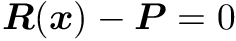Where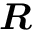is the (possibly non-linear) internal elastic force residual and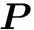is the external force vector (for example, gravitation force or surface traction).

Following the Newton-Raphson method, we pose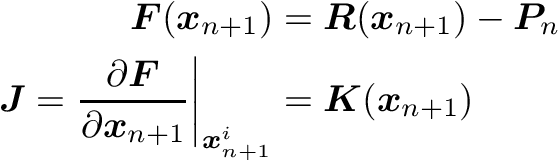where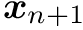is the unknown position vector at the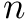th time step. We then iteratively solve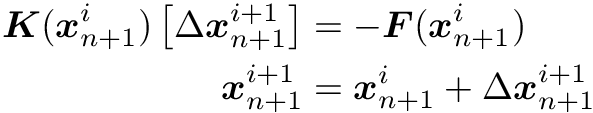#include <StaticODESolver.h>

Inheritance diagram for SofaCaribou::ode::StaticODESolver: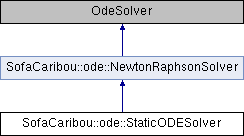## Public Types

template<typename T >
using Data = sofa::core::objectmodel::Data< T >Public Types inherited from SofaCaribou::ode::NewtonRaphsonSolver
template<typename T >
using Data = sofa::core::objectmodel::Data< T >

template<typename T >

## Public Member Functions

SOFA_CLASS (StaticODESolver, NewtonRaphsonSolver)Public Member Functions inherited from SofaCaribou::ode::NewtonRaphsonSolver
SOFA_CLASS (NewtonRaphsonSolver, sofa::core::behavior::OdeSolver)

void init () override

void solve (const sofa::core::ExecParams *params, SReal dt, sofa::core::MultiVecCoordId x_id, sofa::core::MultiVecDerivId v_id) override

auto iteration_times () const -> const std::vector< UNSIGNED_INTEGER_TYPE > &
List of times (in nanoseconds) that each Newton-Raphson iteration took to compute in the last call to Solve().

auto squared_residuals () const -> const std::vector< FLOATING_POINT_TYPE > &
The list of squared residual norms (||r||^2) of every newton iterations of the last solve call.

auto squared_initial_residual () const -> const FLOATING_POINT_TYPE &
The initial squared residual (||r0||^2) of the last solve call.

The documentation for this class was generated from the following files:
• /home/jnbrunet/sources/caribou/src/Plugin/Ode/StaticODESolver.h
• /home/jnbrunet/sources/caribou/src/Plugin/Ode/StaticODESolver.cpp